SSC CGL Previous Year Questions: Geometry- 1

# SSC CGL Previous Year Questions: Geometry- 1 - SSC CGL

Test Description

## 19 Questions MCQ Test SSC CGL (Tier - 1) - Previous Year Papers (Topic Wise) - SSC CGL Previous Year Questions: Geometry- 1

SSC CGL Previous Year Questions: Geometry- 1 for SSC CGL 2023 is part of SSC CGL (Tier - 1) - Previous Year Papers (Topic Wise) preparation. The SSC CGL Previous Year Questions: Geometry- 1 questions and answers have been prepared according to the SSC CGL exam syllabus.The SSC CGL Previous Year Questions: Geometry- 1 MCQs are made for SSC CGL 2023 Exam. Find important definitions, questions, notes, meanings, examples, exercises, MCQs and online tests for SSC CGL Previous Year Questions: Geometry- 1 below.
Solutions of SSC CGL Previous Year Questions: Geometry- 1 questions in English are available as part of our SSC CGL (Tier - 1) - Previous Year Papers (Topic Wise) for SSC CGL & SSC CGL Previous Year Questions: Geometry- 1 solutions in Hindi for SSC CGL (Tier - 1) - Previous Year Papers (Topic Wise) course. Download more important topics, notes, lectures and mock test series for SSC CGL Exam by signing up for free. Attempt SSC CGL Previous Year Questions: Geometry- 1 | 19 questions in 20 minutes | Mock test for SSC CGL preparation | Free important questions MCQ to study SSC CGL (Tier - 1) - Previous Year Papers (Topic Wise) for SSC CGL Exam | Download free PDF with solutions
 1 Crore+ students have signed up on EduRev. Have you?
SSC CGL Previous Year Questions: Geometry- 1 - Question 1

### ΔABC ~ ΔNLM and ar(ΔABC) : ar(ΔLMN) = 4 : 9. If AB = 6 cm, BC = 8 cm and AC =12 cm, then ML is equal to:       (SSC CGL-2018)

Detailed Solution for SSC CGL Previous Year Questions: Geometry- 1 - Question 1

We have,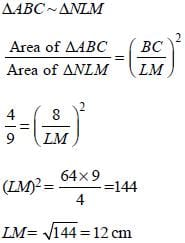SSC CGL Previous Year Questions: Geometry- 1 - Question 2

### In a circle of radius 17 cm, a chord is at a distance of 8 cm from the centre of the circle. What is the length of the chord?       (SSC CGL-2018)

Detailed Solution for SSC CGL Previous Year Questions: Geometry- 1 - Question 2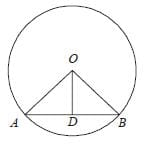Here, AB is a chord
OA = OB = 17cm (radius)
OD = 8cm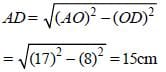Chord AB = 2 ´ 15 = 30cm

SSC CGL Previous Year Questions: Geometry- 1 - Question 3

### In a circle with centre O, an arc ABC subtends an angle of 110° at the centre of the circle. The chord AB is produced to a point P. Then ∠CBP is equal to:       (SSC CGL-2018)

Detailed Solution for SSC CGL Previous Year Questions: Geometry- 1 - Question 3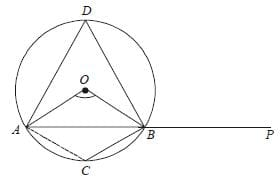Here, OA = OB (radius of the circle).
∴ ∠OAB = ∠OBA
In ΔOAB,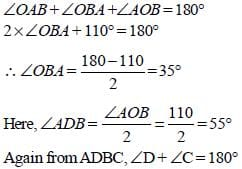∠C = 180° – 55° = 125°
∠CBP = 180° - 125° = 55°

SSC CGL Previous Year Questions: Geometry- 1 - Question 4

In ΔABC, P is a point on BC such that BP : PC = 4 : 11. If Q is the midpoint of BP, then ar(ΔABQ) : ar(ΔABC) is equal to:      (SSC CGL-2018)

Detailed Solution for SSC CGL Previous Year Questions: Geometry- 1 - Question 4Here, BC = BP + PC
= 4x + 11x = 15x
and BQ = PQ = 2x
Let AD is the height of the ΔABC,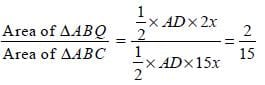SSC CGL Previous Year Questions: Geometry- 1 - Question 5

In ΔABC, AD is the median and G is a point on AD such that AG : GD = 2 : 1. Then ar(ΔBDG) : ar(ΔABC) is equal to:       (SSC CGL-2018)

Detailed Solution for SSC CGL Previous Year Questions: Geometry- 1 - Question 5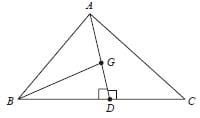Let ΔABC is a equilateral triangle in which AD is a median.
Then, ∠ADB = 90° {∵ ΔABC is equilateral)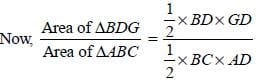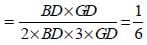SSC CGL Previous Year Questions: Geometry- 1 - Question 6

From a point P outside a cir cle, PAB is a secan t and PT is a tangent to the circle, where, A, B and T are points on the circle. If PT = 5 cm, PA = 4 cm and AB = x cm, then x is equal to:       (SSC CGL-2018)

Detailed Solution for SSC CGL Previous Year Questions: Geometry- 1 - Question 6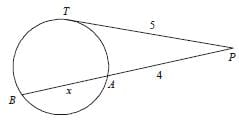From circle properties
PT2 = PA ´ PB
(5)2 = 4 x (x + 4)
x + 4 = (25/4) = 6.25
x = 6.25 – 4 = 2.25 cm

SSC CGL Previous Year Questions: Geometry- 1 - Question 7

ΔABC ~ ΔRQP and AB = 4 cm, BC = 6 cm and AC = 5 cm. If ar(ΔABC) : ar(ΔPQR) = 9 : 4, then PQ is equal to:       (SSC CGL-2018)

Detailed Solution for SSC CGL Previous Year Questions: Geometry- 1 - Question 7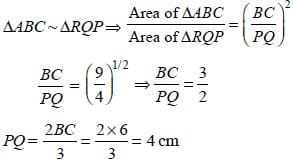SSC CGL Previous Year Questions: Geometry- 1 - Question 8

In a circle with centre O, AB is the diameter and CD is a chord such that ABCD is a trapezium. If ∠BAC = 40°, then ∠CAD is equal to:       (SSC CGL-2018)

Detailed Solution for SSC CGL Previous Year Questions: Geometry- 1 - Question 8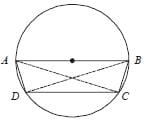As AB is a diameter.
In Δ ABC, ∠BCA = 90°
∠BAC = 40°
∴ ∠ABC = 180° – 90° – 40° = 50°
and ABCD is cyclic trapezium
so, ∠ABC + ∠ADC = 180°
∴ ∠ADC = 180° – ∠ABC
= 180° – 50° = 130°
and ∠DCA = ∠BAC = 40° {∵ AB || CD}.
= 180° – (130° + 40°) = 10°

SSC CGL Previous Year Questions: Geometry- 1 - Question 9

It is given that ΔABC ~ ΔPRQ and that Area ABC : Area PRQ = 16 : 169. If AB = x, AC = y,  BC = z (all in cm), then PQ is equal to:      (SSC CHSL-2018)

Detailed Solution for SSC CGL Previous Year Questions: Geometry- 1 - Question 9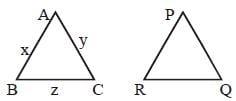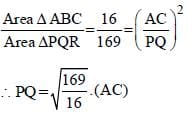= 13/4 y.

SSC CGL Previous Year Questions: Geometry- 1 - Question 10

The side BC of a right-angled triangle ABC (∠ABC = 90º) is divided into four equal parts at P, Q and R respectively. If AP2 + AQ2 + AR2 = 3b2 + 17na2, then n is equal to :       (SSC CHSL-2018)

Detailed Solution for SSC CGL Previous Year Questions: Geometry- 1 - Question 10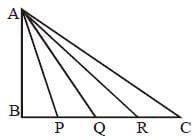In ΔABC, AB = c, BC = a, and AC= b.
and BP = PQ = QR = RC = a/4.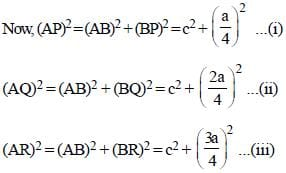from (i) + (ii) + (iii)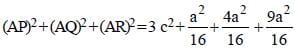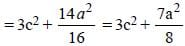Again from ΔABC, b2 = c2 + a2
c2 = b2 – a2
∴ AP2 + AQ2 + AR2 = 3 (b2 – a2) + 7a2/8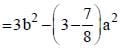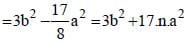Hence, n = - (1/8).

SSC CGL Previous Year Questions: Geometry- 1 - Question 11

OABC is a quadrilateral, where O is the centre of a circle and A, B, C are points in the circle, such that ∠ABC = 120º. What is the ratio of the measure of ∠AOC to that of ∠OAC?       (SSC CHSL-2018)

Detailed Solution for SSC CGL Previous Year Questions: Geometry- 1 - Question 11

Ratio of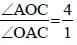SSC CGL Previous Year Questions: Geometry- 1 - Question 12

PA and PB are two tangents from a point P outside a circle with centre O. If A and B are points on the circle such that ∠APB = 80°, then ∠OAB is equal to:       (SSC Sub. Ins. 2018)

Detailed Solution for SSC CGL Previous Year Questions: Geometry- 1 - Question 12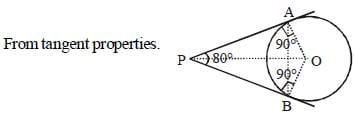∠PAO = 90°
and ∠OPA = 80°/2 = 40°
In Δ AOP, ∠AOP + ∠APO + ∠PAO = 180°
∠AOP + 40° + 90° = 180°
∴∠AOP = 180° – 130° = 50°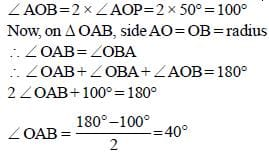SSC CGL Previous Year Questions: Geometry- 1 - Question 13

ABCD is a cyclic quadrilateral such that AB is the diameter of the circle circumscribing it and ∠ADC = 145°. What is the measure of ∠BAC?        (SSC Sub. Ins. 2018)

Detailed Solution for SSC CGL Previous Year Questions: Geometry- 1 - Question 13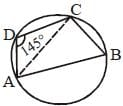As ABCD is a cyclic quadrilateral
∴ ∠B + ∠D = 180°
∠B + 145° = 180°
∠B = 180° – 145° = 35°
Again as AB is a diameter.
∴ ∠ACB = 90°
Now, In ΔACB, ∠ BAC + ∠ABC + ∠ACB = 180°
∠BAC + 35° + 90° = 180°
∠BAC = 180° – 90° – 35° = 55°.

SSC CGL Previous Year Questions: Geometry- 1 - Question 14

In ΔABC, ∠A = 30°. If the bisectors of the angle B and angle C meet at a point O in the interior of the triangle, then ∠BOC is equal to:      (SSC Sub. Ins. 2018)

Detailed Solution for SSC CGL Previous Year Questions: Geometry- 1 - Question 14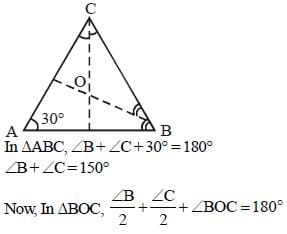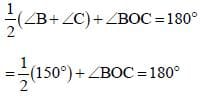= 75° + ∠BOC = 180°
∠BOC = 180° – 75° = 105°

SSC CGL Previous Year Questions: Geometry- 1 - Question 15

Let ΔABC ~ ΔQPR and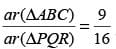. If AB = 12cm, BC = 6cm and AC = 9cm. Then PR is equal to:      (SSC Sub. Ins. 2018)

Detailed Solution for SSC CGL Previous Year Questions: Geometry- 1 - Question 15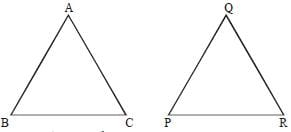As ΔABC ~ ΔQPR, then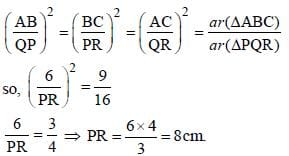SSC CGL Previous Year Questions: Geometry- 1 - Question 16

In the given figure , BD passes through centr e O, AB = 12 and AC = 8. What is the radius of the circle?       (SSC Sub. Ins. 2017)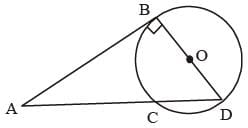Detailed Solution for SSC CGL Previous Year Questions: Geometry- 1 - Question 16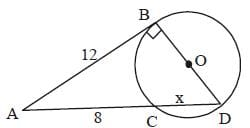According to question,
AB = 12
AC = 8
As, we know that
(12)2 = 8 × (8 + x)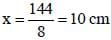ΔABD is a right angle because ∠B = 90°
By Pythagorean theorem,
BD2 = (18)2 – (12)2 {Here AD = 8 + 10 = 18 cm}
= 324 – 144 = 180
∴ BD = 6√5 cm
∴ Radius =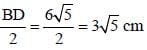SSC CGL Previous Year Questions: Geometry- 1 - Question 17

In the given figure, area of isosceles triangle PQT is 128 cm2 and QT = PQ and PQ = 4 PS, PT || SR, then what is the area (in cm2) of the quadrilateral PTRS?        (SSC Sub. Ins. 2017)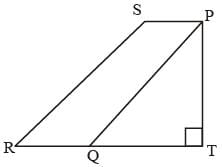Detailed Solution for SSC CGL Previous Year Questions: Geometry- 1 - Question 17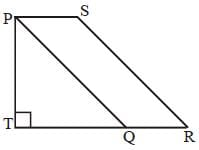From question, PT = TQ = x (Let)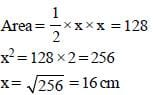Again,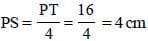As PQ || RS and PS || QR (By symmetry)
∴ PQRS is a parallelogram with base (b) = 4 cm height (h) = PT = 16 cm
Area = b × h = 4 × 16 = 64 cm2

SSC CGL Previous Year Questions: Geometry- 1 - Question 18

If ΔDEF is right angled at E, DE = 15 and ∠DFE = 60°, then what is the value of EF?       (SSC Sub. Ins. 2017)

Detailed Solution for SSC CGL Previous Year Questions: Geometry- 1 - Question 18

According to question,
DE = 15, EF = ?
∠DFE = 60°
∠DEF = 90°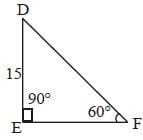∴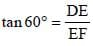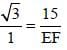∴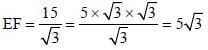SSC CGL Previous Year Questions: Geometry- 1 - Question 19

In the given figure, O is the centre of the circle, ∠DAB = 110°and ∠BEC = 100°. What is the value (in degrees) of ∠OCB?            (SSC Sub. Ins. 2017)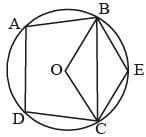Detailed Solution for SSC CGL Previous Year Questions: Geometry- 1 - Question 19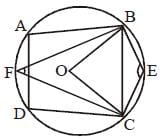∠BEC = 100° (given)
So, ∠BEC + ∠BFC = 180°
100° + ∠BFC = 180°
⇒ ∠BFC = 180° – 100° = 80°
Now, ∠BOC = 2 × ∠BFC (Angle made by the same chord at the center)
= 2 × 80° = 160°
In ΔOBC, OB = OC (radius)
∴ ∠OBC = ∠OCB
Now, ∠BOC + ∠OBC + ∠OCB = 180°
160° + 2∠OCB = 180°
⇒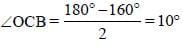## SSC CGL (Tier - 1) - Previous Year Papers (Topic Wise)

250 tests
Information about SSC CGL Previous Year Questions: Geometry- 1 Page
In this test you can find the Exam questions for SSC CGL Previous Year Questions: Geometry- 1 solved & explained in the simplest way possible. Besides giving Questions and answers for SSC CGL Previous Year Questions: Geometry- 1, EduRev gives you an ample number of Online tests for practice

250 tests